# Problems related to parabolas

##1. Overview

The parabola is a special case of a conic (section). Many of its properties discussed in the context of Euclidean Geometry are in reality not metric but projective. A thorough plan of investigation would be to develop first the general theory of projective conics, which doesn't distinguish between ellipses, parabolas and hyperbolas. This theory deals with the intersection properties and has the flavor of algebra. The various kinds of conics are obtained by declaring a projective line as line at infinity and considering the complementary point set. This is the afine plane and there one distinguishes the conics according to the number of points they share with the line at infinity. In fact, in the affine plane there are essentially only one ellipse, one parabola and one hyperbola, all other being afinely equivalent to these three. The distinguished kinds of conics appear after introducing in the afine space a euclidean metric.
So this is not an attempt to organize the whole subject, but simply a compilation of various problems that attracted at times my interest. For the convenience of the reader I include some preliminary propositions on the basic properties of this kind of conics.

##2. Metric properties of Parabola

The parabola is characterized as a curve c whose points P are at equal distances, PF = PA, from a fixed point F (focus) and a fixed line a (directrix).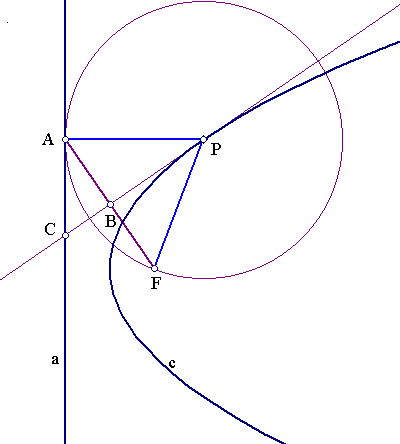It follows that given the focus F and the directrix a of a parabola, one can construct arbitrary many points by the following recipe: 1) Start with a point A on a, 2) Draw the orthogonal AP to a at A, 3) Join A with F and draw the middle-orthogonal to AF, line BF, 4) The intersection point P of the lines BP, AP is on the parabola. The following properties are immediate consequences:
Figure 1 Figure 2 Figure 3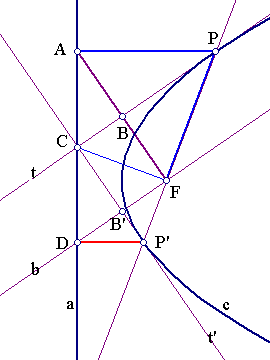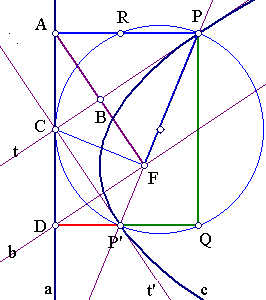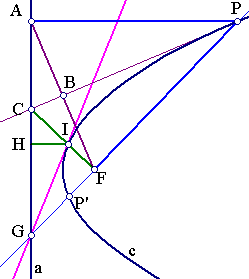1. The tangent t to c at P is the middle-orthogonal line (medial line) of AF. (fig.1)
2. The locus of centers of circles tangent to a fixed line a and passing through a fixed point F is the parabola with directrix a and focus F. (fig.1)
3. Given a fixed line a and a fixed point F, the envelope of all medial lines AF, for points A on a, is the parabola with focus F and directrix a. (fig.1)
4. The line CF is orthogonal to PF and tangent to the circle with radius PF = PA (fig.1 not drawn).
5. As A moves on a, the middles B of AF describe a line b parallel to a at x/2, where x (called parameter of the parabola) is the distance of the focus from the directrix a . The line b is tangent at the vertex of the parabola. The vertex is the nearest to the directrix point of the parabola. (fig.1 not drawn)
6. Draw from F a parallel b to PC and from C a parallel t' to FA. t' is again a tangent to the parabola. (fig.2)
7. The tangents to the parabola from a point C on the directrix form a right angle at C. (fig.2)
8. The line through the tangent points P, P' passes through the focus F. (fig.2)
9. C is the middle of AD. The quadrangles APFC and DP'FC are similar. CF2 = AP*DP'. (fig.2)
10. The circle with diameter PP' touches the directrix a at C. (fig.2)
11. AR = DP' and APQD is a rectangle. (fig.2)
12. Let G be the intersection point of the directrix a with PP'. Let I be the intersection point of CF with the parabola c. The tangent to c at I passes through G. (fig.3) (The triangles GIH and GIF are equal.)
13. GI is the bisector of the angle AGP. (fig.3)
14. Consider a fixed line a and a fixed point F. For every point G on a let GI be the bisector of the angle between the line a and GF. The envelope of the lines GI is the parabola with directrix a and focus F. (fig.3)
15. All quadrangles APFC are bicircular i.e. they have inscribed and circumscribed circles. The center of the inscribed circle is the intersection point of PC and GI.

##3. Chords of Parabolas

Consider a chord PP' of a parabola and the tangents at its endpoints intersecting at D. We remark:
Figure 1 Figure 2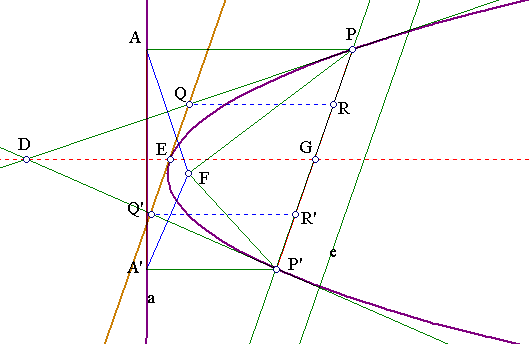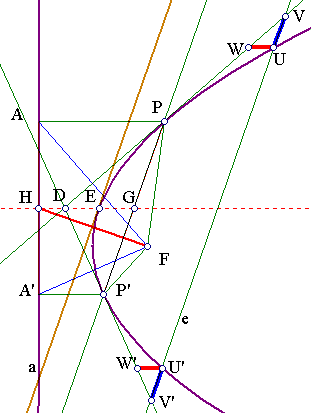1. The line DG joining D to the middle G of PP' is parallel to the axis of the parabola. (fig.1)
2. The intersection point E of the parabola with DG is the middle of DG. (fig.1)
3. The tangent at E is parallel to the chord PP' and QQ' is the half of PP'. (fig.1)
4. The middle points G of all chords PP', parallel to a given direction e are on a line DG parallel to the axis of the parabola. (fig.1)
5. The previous line DG is the parallel to the axis going through the tangent point E with a line parallel to e.
6. The line HF is orthogonal to the chord direction. (fig.2)
7. The segments on the chord UV, U'V' between the parabola and the tangents are equal. (fig.2)
8. The segments UW, U'W' are also equal. (fig.2)
Proof hints
1. Because PD, PD' are medial lines of AF, A'F hence D is the circumcenter of the triangle AFA'. D projects on the middle of AA'.
2. Apply (1) to the tangent pairs QE, QP and Q'E, Q'P'. R, R' are the middles of GP, GP' hence QQ' joins the middles of PD, DP'.
3. Follows from (2).
4. DG passes through the fixed point E, where the tangent is parallel to the direction e.
5. See above.
6. The tangent at E is medial line of HF.
7. UU' and VV' have a common point as their middle.
8. Follows from the previous.

##4. Triangles tangent to parabolas

Consider a triangle ABC, formed by the tangents at a parabola at its point P, Q, R. The following properties relate the geometry of the triangle to that of the parabola.
Figure 1 Figure 2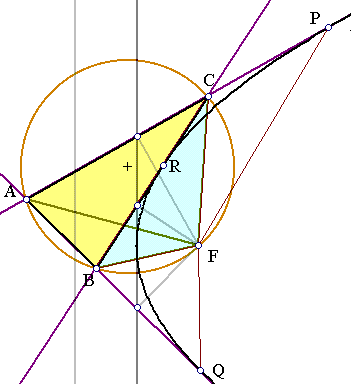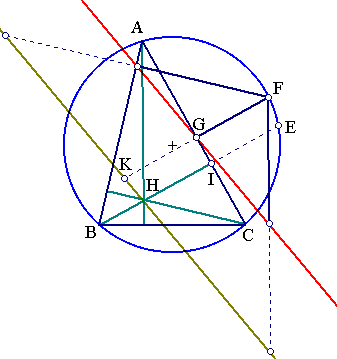1. The projections of the focus on the sides of the triangle lie on the tangent at the vertex of the parabola.
2. The previous tangent is a Simson line of the triangle.
3. The focus F lies on the circumcircle of the triangle.
4. Consider the two tangents fixed, say AC and AB, and the third BC moving (R moving on c). The triangle BFC remains similar to itself.
5. This triangle as R converges to P takes the place of AFP. Similarly as R converges to Q it takes the place of AFQ. Thus the three triangles are similar.
6. A triangle BFC with fixed F changes by remaining similar to itself and moving B on a fixed line AC. Then its side BC envelopes a parabola with focus F.
7. The orthocenter of ABC lies on the directrix of the parabola (Steiner I, p. 134, Lehrsatz 5).
Proof hints
1. Restatement of a property of the focus, see 2-5.
2. Characteristic property of Simson lines.
3. Because its projections on the sides are on a line.
4. Measure angles of the inscribed quadrangle ABFC.
5. Obviously. triangle(BFC) ~ triangle(AFP) ~ triangle(QFA).
6. The other corner C moves on a fixed line AC. This is true for every point X moving with BFC but remaining at fixed distances from its sides (relatively fixed to BFC). Thus, reduce the problem to the previous one.
7. This is a property of the doubled Simson line in triangles. By this I mean the line parallel to the Simson through the symmetric of the point F (fig.2) w.r. to the Simson line. In fact, let E be the symmetric of the orthocenter H w.r to a side of the triangle and lying on the same arc with F, say AC. Reflect the line EF w.r. to AC. The reflected line coincides with the doubled Simson. Hence later passes through H.

##5. All parabolas tangent to a triangle

Consider a triangle ABC and find all parabolas tangent to all three sides of the triangle. The problem is related to the Simson lines of the triangle through the last proposition on the doubled Simson line. In fact, every such parabola has its directrix passing through the orthocenter as the doubled Simson line of a point on the circumcircle which is the focus of the parabola. Thus to determine all such parabolas one can follow one of these recipes, which construct the parabola from its directrix (a) or from its focus (b):
a) Draw a line a through the orthocenter and find the point F on the circumcircle for which a is the corresponding doubled Simson line.
b) Start with a point on the circumference F and find the corresponding doubled Simson line a.
The first method shows that to every direction corresponds one parabola tangent to the triangle having this direction as its directrix. The second method shows that to every point on the circumcircle corresponds one parabola tangent to the triangle having that point as its focus. The following exercises relate to these constructions.
Figure 1 Figure 2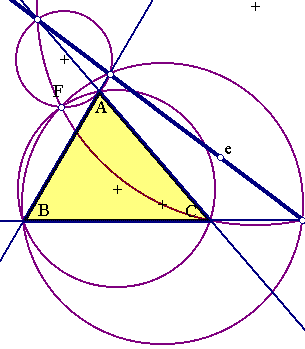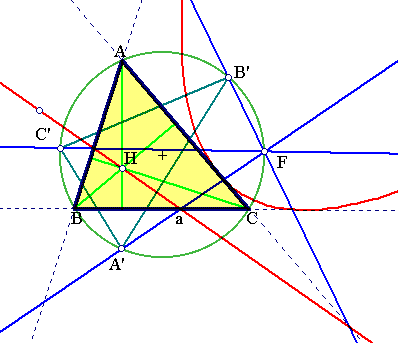1. The focus of a parabola tangent to four lines is the Miquel point of the lines: the common point of the circumcircles of the four triangles formed by the four lines.
2. The orthocenters of the above four triangles are on a line (Steiner I p. 128, Aufgabe 8), the directrix of the corresponding parabola.
3. The projections of F on the four lines are on a line, which is a simultaneous Simson line for the four triangles.
4. There is exactly one parabola tangent to four lines.
5. Let a be a line through the orthocenter H of ABC. The reflected lines of a w.r. to the sides pass through a common point F, lying on the circumcircle. The Simson line of F is a. (Steiner I. p. 128, Aufgabe 6).
6. The same lines pass through the symmetrics A', B', C' of H on the sides.
7. The triangle A'B'C' is similar to the orthic and the angles at F are equal or complementary to those of the orthic triangle.
Proof hint
Start from the last proposition. The angles at F of the reflected lines can be easily computed and shown to coincide with the respective of the orthic triangle.
Note that the parabola is tangent at the line at infinity so four real tangents plus the line at infinity are five tangents determining completely a conic.

##6. Kiepert's parabola of a triangle

From all parabolas tangent to the sides of a triangle, Kiepert's one is distinguished by having as directrix the Euler line of the triangle. It is also characterized by being simultaneously tangent to the Lemoine axis g of the triangle which is the medial line of the segment JJ' defined by the isodynamic points of the triangle.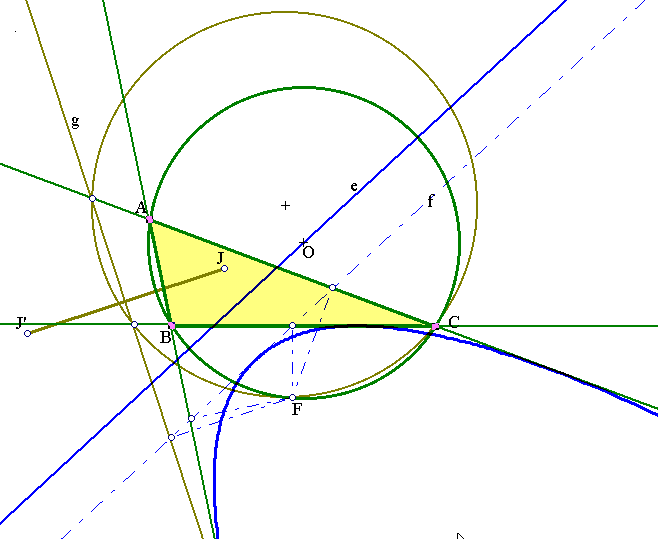The construction of the parabola can be done by using the results 5-1 to 5-4.

##7. Orthic Parabolas of a triangle

Consider a triangle ABC and a point P moving on AC. From P draw the orthogonals to the sides cutting them at U, V. The line UV, as P moves along AC, envelopes a parabola with focus the foot F of the altitude from B and directrix the line through the other feet of the altitudes I, K.
This is proved in a series of remarks, starting with figure 2 below.
Figure 1 Figure 2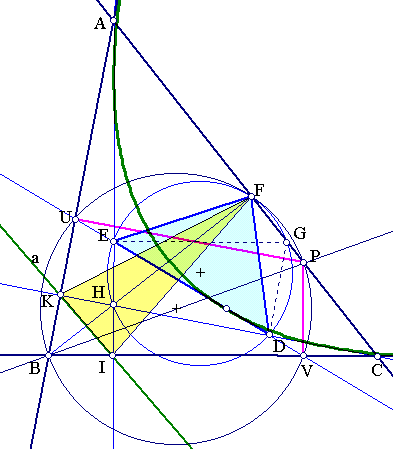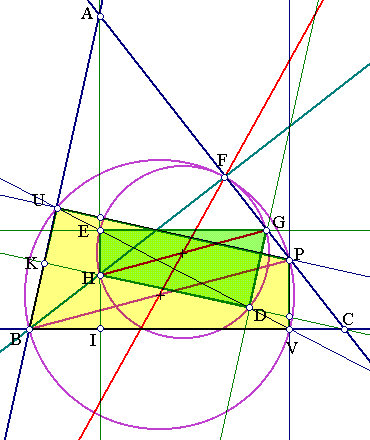1. Let D, E the intersections of UV with the altitudes CK, AI. At D, E draw DG, EG orthogonal to these altitudes intersecting at G. H being the orthocenter GEHD is similar to the quadrangle PVBU.(fig.2)
2. In fact, DEG ~ UVB and EHD ~ VPU the similarity ratio for both triangle pairs being r=ED/UV.
3. The ratio of the parallel segments HG/BP being also r implies that G is on AC and their similarity center is on F, foot of the altitude from B.
4. The circles inscribing the quadrangles GEHD and PVBU, having respective diameters HG and BP are tangent at F.
5. Pass now to fig.1. The triangle FED is similar to the original ABC.
6. In fact, the angles are: ang(EFD) = ang(B), ang(FED) = ang(FHD) = ang(BIK) = ang(A).
7. The triangle FED remains similar to ABC, has fixed F and D moves along CH hence (4.6) ED envelopes a parabola.
8. FIC and FAC are two particular positions of FED, showing that the parabola is tangent to the sides BA, BC.
9. The directrix of the parabola contains the symmetric of F w.r. to HC hence lies on the side KI of the orthic triangle (having the altitudes as bisectors).
10. Similarly the triangle UFV remains similar to itself and the same parabola envelopes UV.

##8. Parabolas enveloping lines

There is a general recipe to generate parabolas, which envelope lines. The method uses two fixed lines b,c on which a third, variable one a, cuts points such that x = AC, y = AB satisfy a linear relation kx + my + n = 0, for some fixed constants k, m, n. Then line a envelopes a parabola which is tangent to the lines b,c. (fig.1)
Figure 1 Figure 2 Figure 3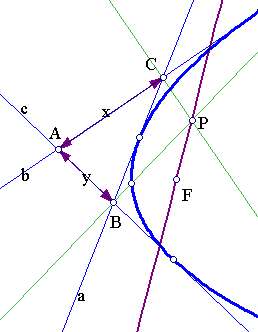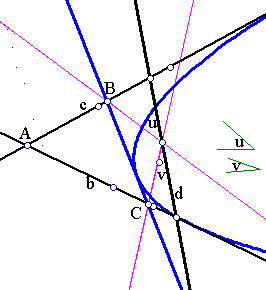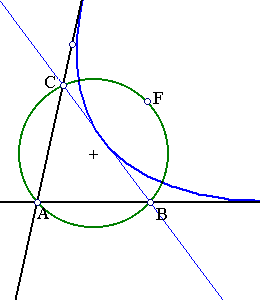The statement above as well as the two applications below are proved by straightforward calculations.
1. Show that the orthogonals to the lines b, c at C and B, respectively, meet at a point P which describes a line as c varies in the way indicated above.(fig.1).
2. Thus, by the previous paragraph BC envelopes a parabola.
3. Given three fixed lines b, c and d and a moving point Q on d, draw two lines a', b' from Q at fixed angles to d, which cut c, b at B, C respectively (fig.2) show that BC envelopes a parabola (generalization 7-10 above).
4. Given again the fixed lines b, c and a fixed point F, draw variable circles going through A and F. Let B, C be respectively the other intersection points with lines c and b (fig.3). Show that BC envelopes a parabola, whose focus if F.
For the two last propositions suffices to apply the first after showing that the variable line BC cuts on the fixed one two segments satisfying the relation kx + my + n = 0, for some fixed constants k, m, n.

##9. Additional Exercises

1. The submnormal QR (projection on the axis of the normal segment PR) of a point P on the parabola is constant and equal to the parameter BF (fig.1).
2. If a parabola is tangent to the sides of an equilateral triangle, then the lines joining the vertices and the points of tangency of the opposite sides pass through the focus. (Steiner I, p. 134, Lehrsatz 6) (fig.2)
3. Show that the area of the parabola sector BCD inside the tangent triangle ABC is (2/3)s, where s is the area of the tangent triangle ABC. (fig.3)
Figure 1 Figure 2 Figure 3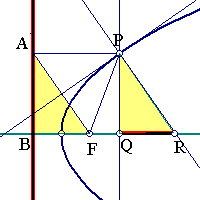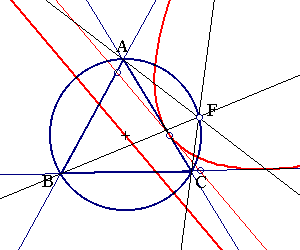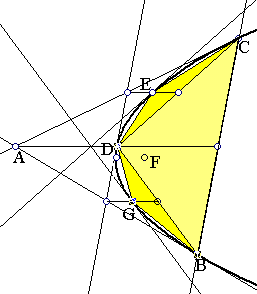1. PR is the normal at P and the triangles ABF and PQR are equal.
2. From pascal's theorem these lines pass always through a common point. The proposition follows from the fact that the angles at F are all 60 degrees and AFC remains similar to itself as AC turns around F (4-4). So these common point coincides with the focus F.
3. Follow Archimedes method by writing the area as an infinite sum of the areas of triangles BDC, DCE etc. forming a geometric series of ration 1/4. Use the results of third paragraph.

##10. On a problem of Klaoudatos

N. Klaoudatos presented, in his talk on the ICTM2 conference, the solution of a problem that I will formulate in a slightly more general form than his. I will indicate a solution not involving explicitly parabolas, as was the case with his solution.
Problem
A point P moves on the basis BC of a triangle ABC. Lines PD, PE at fixed angles a(BPD), b(CPE) from P are drawn to the sides of angle A. Find the position P for which DE becomes minimum.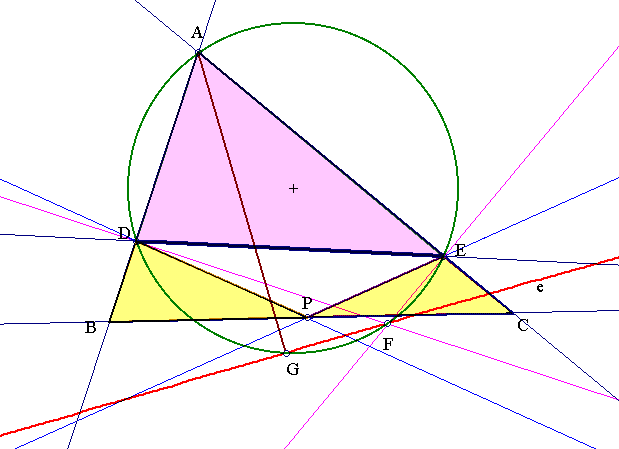Consider the orthogonals to the sides of the angle A at the points D, E: DF and EF, intersecting at F. As P moves on the line BC, the point F moves on another fixed line e. This can be shown by using the fact that the small triangles BPD and CEP remain similar to themselves as P moves on BC. Thus the problem is reduced to find the minimum of DE, while F moves on the fixed line e and is projected orthogonally at D and E. This is a simple problem having as solution the projection G of A on e.

##11. Variations on the previous problem

A point P moves on the basis BC of a triangle ABC. Lines PE, PF at fixed angles u(APE), v(APF) to AP from P are cutting the sides of A at E, F. Find the position P for which EF becomes minimum. (16-01-2013 update) This has been answered by Mehmet Kilic . See Kilic Solution .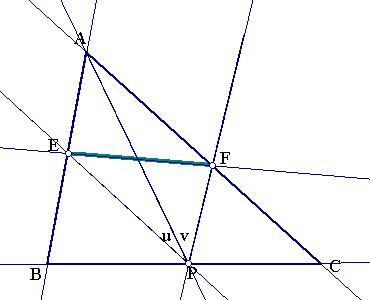Remarks Some similar problems are to find the position of P, for which:
1. the segment AE becomes a minimum
2. the segment AF becomes minimum ( The two points do not coincide )
3. the sum AE+AF becomes minimum
4. the perimeter/area of AEF becomes minimum
5. the perimeter/area of the triangle PEF becomes a minimum
6. the sum x(P) = area(AEF)+area(PEF) becomes a minimum
All of these points, where the minimum takes place, depend on u, v. Thus, one can ask: Are there values of u, v, for which all (or some of) these minima occur at the same P?